### AVERAGEA Function Examples – Excel & Google Sheets

This tutorial demonstrates how to use the Excel AVERAGEA Function in Excel to calculate the average ( mean ).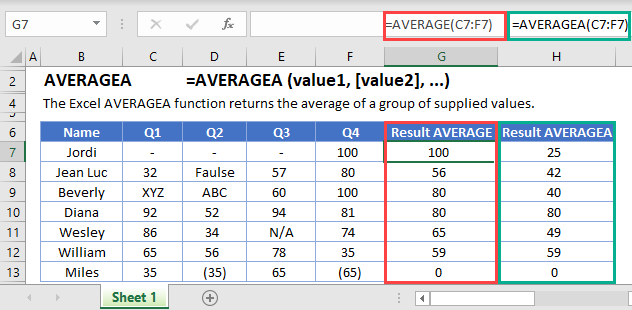AVERAGEA Function Overview
The AVERAGEA Function Averages a series. Blank values are ignored. Text and and the logical value FALSE are treated as 0. The logical value TRUE counts as 1.

To use the AVERAGEA Excel Worksheet Function, select a cell and type: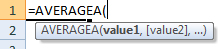(Notice how the formula inputs appear)

## AVERAGEA function Syntax and inputs:

array – An array of numbers.

# What is the AVERAGEA Function?

The Excel AVERAGEA Function gives you the average (arithmetic mean) of a range of values. The values can include numbers, cell references, cell ranges, constants, or arrays.

AVERAGEA is very similar to the AVERAGE function, but with a few key differences:

• AVERAGEA counts cells containing text as 0. AVERAGE ignores them
• AVERAGEA evaluates Boolean values. It counts TRUE as 1, and FALSE as 0. AVERAGE ignores Booleans.

## What is the arithmetic mean?

The arithmetic mean, usually just called the mean, is the sum of a set of numbers, divided by how many numbers appear in the set.

As a simple example, take the numbers 1,2,3,4,5. If we add all these together, we get 15. And we’ve got five numbers in the set, so the mean is 15 / 5 = 3.

AutoMacro - VBA Code Generator

## How to Use the AVERAGEA Function

Here’s how you use the Excel AVERAGEA Function: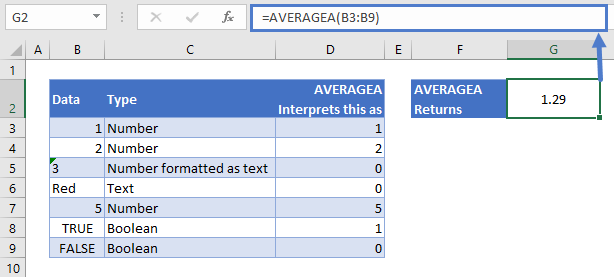Our data is in cells B3:B9, column C describes what type of data it is, and in column D I’ve just shown you how AVERAGEA interprets every value in our range.

From top to bottom:

• B3 and B4 are numbers. AVERAGEA takes these are they are.
• B5 contains a number formatted as text. Note that AVERAGEA does NOT take the numerical value here. Any cells containing text are treated as 0.
• B6 is another example of a text cell.
• B8 contains TRUE. AVERAGEA interprets this as 1.
• B9 contains FALSE. AVERAGEA interprets this as 0.

So although we’ve presented our data in a host of different formats here, AVERAGEA will first convert these to the numbers as shown in column D, and then calculate the mean.

If you don’t want to count cells containing text, or Boolean values at all, use the AVERAGE Function <<link>> instead.

Note that if any of the cells in the range contain an error, AVERAGEA will also return an error.

## Blanks Vs. Zeros

AVERAGEA ignores cells that are truly blank – that is, they contain no data or formulas whatsoever. However, it does count cells containing 0.

See the example below: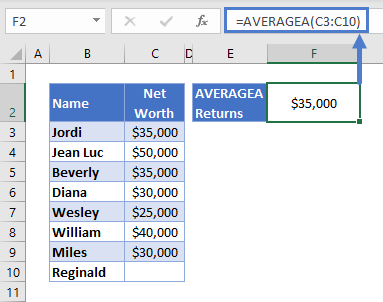The table lists the net worth of a group of work colleagues. We don’t know Reginald’s net worth yet, so that cell is blank. AVERAGEA ignore that cell completely.

If Reginald later supplies his net worth figure, and it’s 0, AVERAGEA will now include him when calculating the mean: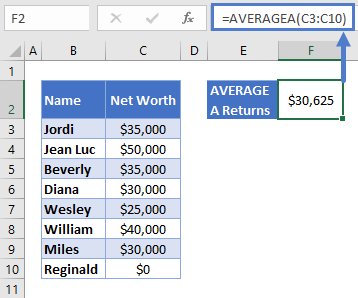Note that if a cell contains a formula that returns an empty string, such as this: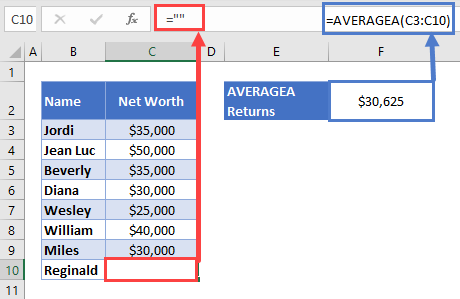AVERAGEA will treat these as any other text, and count it as 0.

## Extreme Values

The arithmetic mean is useful for data where the numbers are relatively close to each other. However, AVERAGEA can give inaccurate estimates if your data contains extreme values – that is, values that sit way outside the rest of the data. Such values are often called “outliers”.

For example, imagine if the team above hired a new employee, Bill Gates: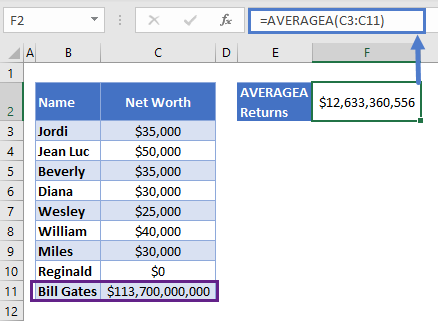Bill Gates is very much an outlier here, and his immense net worth is skewing the mean in his direction. To say that this group has an average net worth of over \$12bn doesn’t tell the story of the data.

If your data contains outliers, consider using Excel’s MEDIAN Function <<link>> instead.

## Calculating the Mean While Ignoring Text Strings and Booleans

If your data contains a lot of cells containing text or TRUE/FALSE values, and you want to get the average but ignore these other cells, use AVERAGE instead.

See the example below: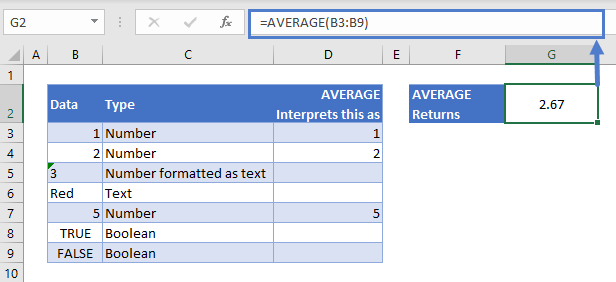This is the same example we looked at earlier, but seen through the eyes of AVERAGE:

• B3 and B4 contain numbers. AVERAGE interprets them as such.
• B5 and B6 contain text. AVERAGE ignores them.
• B7 is another number. AVERAGE includes it.
• B8 and B9 are Booleans, AVERAGE ignores these too.

So the final calculation is:

## AVERAGEA in Google Sheets

The AVERAGEA Function works exactly the same in Google Sheets as in Excel: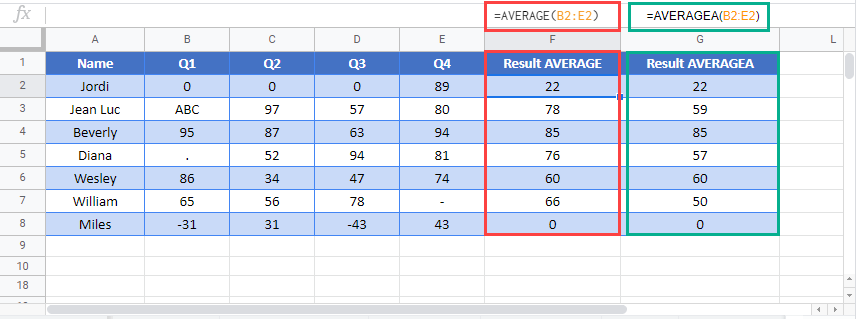## Excel Practice Worksheet

Practice Excel functions and formulas with our 100% free practice worksheets!

• Automatically Graded Exercises
• Learn Excel, Inside Excel!